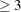# The PRINCOMP Procedure

### ODS Graphics

Statistical procedures use ODS Graphics to create graphs as part of their output. ODS Graphics is described in detail in Chapter 21: Statistical Graphics Using ODS.

Before you create graphs, ODS Graphics must be enabled (for example, by specifying the ODS GRAPHICS ON statement). For more information about enabling and disabling ODS Graphics, see the section Enabling and Disabling ODS Graphics in Chapter 21: Statistical Graphics Using ODS.

The overall appearance of graphs is controlled by ODS styles. Styles and other aspects of using ODS Graphics are discussed in the section A Primer on ODS Statistical Graphics in Chapter 21: Statistical Graphics Using ODS.

Some graphs are produced by default; other graphs are produced by using statements and options. You can reference every graph produced through ODS Graphics by name. The names of the graphs that PROC PRINCOMP generates are listed in Table 91.3, along with a description of each graph and the required statements and options.

Table 91.3: Graphs Produced by PROC PRINCOMP

ODS Graph Name

Plot Description

Statement and Option

PaintedScorePlot

Score plot of component i versus component j, painted by component k

PLOTS=SCORE when number of variablesPatternPlot

Component pattern plot

PLOTS=PATTERN

PatternProfilePlot

Component pattern profile plot

PLOTS=PATTERNPROFILE

ScoreMatrixPlot

Matrix plot of component scores

PLOTS=MATRIX

ScorePlot

Component score plot

PLOTS=SCORE

ScreePlot

Scree and variance plots

Default and PLOTS=SCREE

VariancePlot

Variance proportion explained plot

PLOTS=SCREE(UNPACKPANEL)